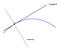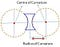“Esoteric” art of 3D Shape Analysis — Level 1 — Differential Geometry

Thus far we have talked about

• the motivation for Shape Analysis — uses in Geometric Deep Learning, understanding properties of 3D shapes and objects, 3D mesh reconstruction from point clouds, etc;
• some basic ‘have-to-know’ operations on shapes — Shape Preserving operations (Translation, Rotation and Scaling) and Non-Shape Preserving operations (Bending and Stretching);
• setting things up with tensorflow-graphics;

And Now … Differential Geometry

Trust me, it’s not as complicated as it sounds.

Differential Geometry is just the use of Calculus to study Geometry problems.Simple Differential Calculus to determine tangent and normal at a Point on Curve.

I will present equations with simple calculus in them, but I feel like you should be able to ignore it and still get a good understanding of the concepts.

But first, why do we even need this information?. But when you look at a surface, say a table or a cereal bowl, what are some of the questions we ask of the surface?

• Is the surface flat or curved? You would expect to have a flat table as opposed to a curved table.
• If it is curved, what is the curvature? Cereal bowls come in different shapes. Some more rounded than others. The flatter a cereal bowl gets, well … it transitions into a plate.
• What is the front of the surface? We put objects on the table, but what does on, not on, above, and below the table actually mean?
• What is the direction of ascent or descent to walk on the surface? Think of pouring milk into the bowl. The minute that milk hits the surface, it travels down to the middle of the bowl. That is, the milk descends down the bowl.

To answer these questions, we will define three terminologies in this article: Tangent, Normal, Curvature. Differential Geometry provides us with the toolbox 🧰 to calculate these.

Parametric Surfaces

Surfaces in real world are continuous. So ideally, we would like to represent these real world object with continuous equations. Enter Parametric Surfaces.

Parametric Curve

But first a slight, yet very relevant, detour. Why not go down 1 dimension and look at just 2D surfaces. That is, let us analyze curves first.

Let’s consider, for example, my fictitious friend Kay is coming over and I know that it takes him 40 minutes to drive a 10 mile twisted-curvy road down to my place. At some random time before he reaches, I give him a call to ask him for an update. To that he responds, “I will be there in 10 minutes”. In other words, Kay has travelled for about 30 minutes out of the 40 minutes of estimated travel time. Which means “he is 7.5 miles through with his Journey”.

We were able to estimate the distance travelled using the knowledge of time. This is called re-parameterization because I used the parameter of time to find Kay’s position on the curve that connects his home to mine. In other words, we parameterized the arc-length of the curve.

Arc-Length of a curve is the distance between two points on curve. AND I MEAN DISTANCE, NOT DISPLACEMENT!

Mathematically we can capture this as follows;

NOTE: You can also say that each point is 3D. That is, each point on our curve would be represented as x, y and z. But because of our parameter space is 1-D, this is still called a curve.

Now suppose, instead, I ask Kay how much more distance he has left to cover before he reaches my place. And that he responds with “I have to drive 2 more miles to get there”, we can estimate that he needs “8 more minutes to reach his destination”

This is the inverse process of being able to estimate time using the knowledge of distance travelled. That is, we were able to map back from arc-length to a parameter value, in this case time.

Once again, the mathematical way of saying this would be as follows;

Just for further clarity and to show off my iPad Pro skills, let me try and capture this in a clean diagram.

Diffeomorphism

In our mathematical equation of re-parameterization, I introduced a function ‘gamma’. In our example, this gamma is a straight line with 0 as the starting time and 40 minutes as the ending time. Also in our example, we were able to invert the process by simply taking the inverse of gamma.

Diffeomorphism is when a parameterization function gamma that is bijective (the mapping is unique between the curves) and both itself and it’s inverse is differentiable (in other words, continuous)

In simpler terms for us common folks, it means that each value of the parameter that we wish to map, should have a unique spot on the curve and likewise each spot on the curve should have a unique value that we can map back to. No two parameter values should map to the same location on the curve and like-wise a point on the curve should not track back to multiple parameter values.

Diffeomorphism is super important. A curve X, and another curve X’, that is obtained after re-parameterization using gamma, are essentially the same when it comes to shape analysis. The two shapes X and X’ are said to be Diffeomorphic.

… back to Parametric Surface

Let’s go ahead and add that third dimension back in. We can extend our understanding of parametric curves to surfaces by simply one more dimension to our parameter space.

That is, a point on the surface can be uniquely identified by 2 numbers (u, v) as opposed to 3 numbers that represent the location of the point in 3D space.

Phheeeeewwww … now that we have a way to represent surfaces in 3D using continuous parametric surfaces, we can finally start talking about tangents and normals again.

Tangent

The tangent is simply the direction of ascent at a specific point on a curve or a surface. The direction 180 degrees opposite the direction of ascent would naturally be the direction of descent, which is also the tangent.

The first differential of the parametric curve (or a parametric surface) gives us the parametric equation of the tangent. Here is a neat article by Andrew and Jimin that explains a little more about the tangent and the process of finding a tangent to a simple curve.

In short, the direction of change within a tiny segment on the curve is the tangent vector at that location.

For a surface however, the tangent vector (T) lies on a plane spanned by the partial differential of the surface with respect to each dimension.

Normal

The normal is the direction perpendicular (or orthogonal) to the Tangent. If you stand on the surface, normal is the direction of looking up from the surface.

To determine the normal we just differentiate the parametric equation for the Tangent. At first, this isn’t really intuitive. But if you think about differentiation as the rate of change of we can explain things better.

The diagram below should help foster this intuition. A more mathematical proof is presented by Paul Dawkin’s online notes.

Another way to look at normal: Normal is the direction of change of tangents at two nearby locations.

The non-differential geometry way of doing this is given below (we use simple vector cross-product):

Curvature

When you are driving a car you will notice that some turns don’t require you slow down to turn because they are not tight, but some turns will require you to come close to a full stop before making a turn because these turns are tight.

This concept of tightness of a turn is essentially given by a number called Curvature. A zero-curvature surface is flat. Higher the curvature, tighter the turn.

Curvature can be computed as the magnitude of the vector obtained by double differentiating the parametric curve.

And if you are thinking, “wait … we double differentiated to get the normal too”, then you are right. I like to think of the Curvature as the magnitude of the Normal Vector, not strictly speaking (I say so because normal is a unit vector).

This tightness of a turn can also be represented by another number called Radius of Curvature. It is inversely proportional to the Curvature.

The big idea behind Radius of Curvature is that you can fit a circle (or sphere in the case of surfaces) of some radius that perfectly captures each turn as shown in the image below. Lower the radius, tighter the turn. An infinite radius implies a flat surface.Image taken from this Quora Post. Follow along for an interesting discussion on the difference between Curvature and Radius of Curvature.

Tensorflow Example

Before I wrap up here, I have walked through a couple of examples on how to build a parametric surface. The first surface is a simple sphere as warm up and then we step it up with a 2–3 Trefoil Knot Surface that demonstrates re-parameterization of a Torus Surface. If you please, you could experiment constructions of 3, -7 torus surface and such! Have FUN!!!

Here are few other examples of Parametric Surfaces that maybe built

Alright Alright, where do we go from here?

That was probably a lot to take in. And I know so, because that was lot to write 😛. But we are still working our way up towards understanding shapes. Getting into 3D Graphics and Reconstruction isn’t that easy huh?

In our next article, we are going to continue talking about curvatures for a surface. There are multiple definitions of curvatures for a surface: Normal Curvature, Principal Curvature, and Gaussian Curvature. But to talk more about these we will need to learn about something called the First Fundamental Form and the Second Fundamental Form as well.

If space permits, we will also start defining Topologies and Manifolds, including Hausdorff Spaces and Riemann Manifolds.

Stay tuned for COOLER STUFF PEOPLE!!!

Fight On!

Written by

Kishore Venkateshan

Website: http://kishorevenkateshan.com GitHub: https://github.com/kv3n A Mathematician, Software Engineer and AI Practitioner

Welcome to a place where words matter. On Medium, smart voices and original ideas take center stage - with no ads in sight. Watch
Follow all the topics you care about, and we’ll deliver the best stories for you to your homepage and inbox. Explore
Get unlimited access to the best stories on Medium — and support writers while you’re at it. Just \$5/month. Upgrade